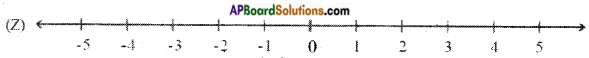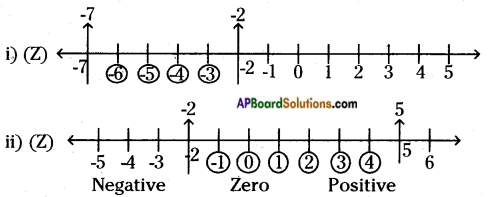AP State Syllabus AP Board 6th Class Maths Solutions Chapter 4 Integers Ex 4.1 Textbook Questions and Answers.

## AP State Syllabus 6th Class Maths Solutions 4th Lesson Integers Ex 4.1

Question 1.
Write True or False against each of the following statements.
i) -7 is on the right side of -6 on the number line.
ii) Zero is a positive number.
iii) 29 is on the right side of zero on the number line.
iv) -1 lies between the integers -2 and 1.
v) There are nine integers between -5 and +5.
i) False
ii) False
iii) True
iv) True
v) TrueQuestion 2.
Observe the following number line and answer the following questions.i) Which is the nearest positive integer to -1?
ii) How many negative numbers you will find on the left side of Zero?
iii) How many integers are there in between -3 and 7?
iv) Write 3 integers lesser than -2.
v) Write 3 integers more than -2.
i) +1 is the nearest positive integer to -1.
ii) On the given number line negative numbers to left of zero are -1, -2, -3, -4, -5.
So, there are 5 in number.
iii) On the number line integers between -3 and 7 are -2, -1, 0, 1, 2, 3, 4, 5, 6.
So, there are 9 in number.
iv) Integers less than -2 means numbers left side of -2.
They are -3, -4, -5, -6, -7, ………
So, 3 integers lesser than -2 are -3, -4, -5.
v) Integers more than -2 means numbers right side of -2.
They are -1, 0, 1, 2, 3, 4, ……..
So, 3 integers more than -2 are -1, 0, 1.Question 3.
Represent the integers on a number line as given below.
i) Integers lies between -7 and -2.
ii) Integers lies Between -2 and 5.